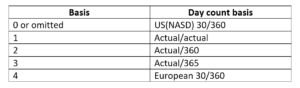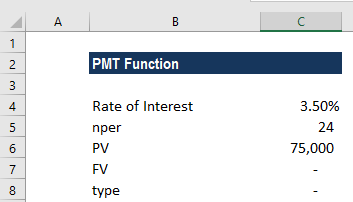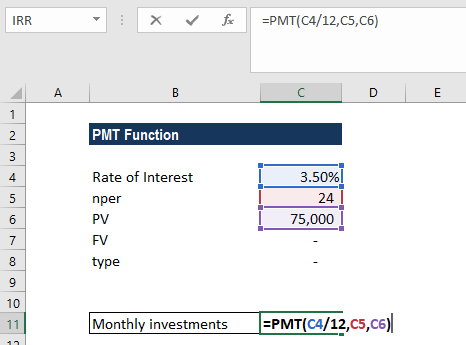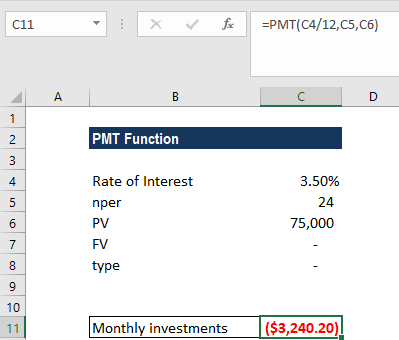# PMT Function

Calculates the payment for a loan or investment with constant payments and fixed interest rate

## What is the PMT Function?

The PMT function is categorized under financial Excel functions. The function helps calculate the total payment (principal and interest) required to settle a loan or an investment with a fixed interest rate over a specific time period.

### Formula

=PMT(rate, nper, pv, [fv], [type])

The PMT function uses the following arguments:

1. Rate (required argument) – The interest rate of the loan.
2. Nper (required argument) – Total number of payments for the loan taken.
3. Pv (required argument) –  The present value or total amount that a series of future payments is worth now. It is also termed as the principal of a loan.
4. Fv (optional argument) – This is the future value or a cash balance we want to attain after the last payment is made. If Fv is omitted, it is assumed to be 0 (zero), that is, the future value of a loan is 0.
5. Type (optional argument) – The type of day count basis to use. The possible values of the basis are:### How to use the PMT Function in Excel?

As a worksheet function, the PMT function can be entered as part of a formula in a cell of a worksheet. To understand the uses of PMT, let us consider an example:

#### Example 1

Let’s assume that we need to invest in such a manner that, after two years, we’ll receive \$75,000. The rate of interest is 3.5% per year and the payment will be made at the start of each month. The details are:The formula used is:We get the results below:The above function returns PMT as \$3,240.20. This is the monthly cash outflow required to realize \$75,000 in two years. In this example:

• The payments into the investment are on a monthly basis. Hence, the annual interest rate is converted to a monthly rate. Also, we converted the years into months: 2*12 = 24.
• The [type] argument is set to 1 to indicate that the payment of the investment will be made at the beginning of each period.
• As per the general cash flow convention, outgoing payments are represented by negative numbers and incoming payments are represented by positive numbers.
• As the value returned is negative, it indicates an outgoing payment is to be made.
• The value 3,240.20 includes the principal and interest but no taxes, reserve payments, or fees.

### A few things to remember about the PMT Function:

1. #NUM! error – Occurs when:
1. The given rate value is less than or equal to -1.
2. The given nper value is equal to 0.
2. #VALUE! error – Occurs when any of the arguments provided are non-numeric.
3. When calculating monthly or quarterly payments, we need to convert annual interest rates or the number of periods to months or quarters.
4. If we wish to find out the total amount that was paid for the duration of the loan, we need to multiply the PMT as calculated by nper.

Thanks for reading CFI’s guide to important Excel functions! By taking the time to learn and master these functions, you’ll significantly speed up your financial analysis. To learn more, check out these additional CFI resources:

• Excel Functions for Finance